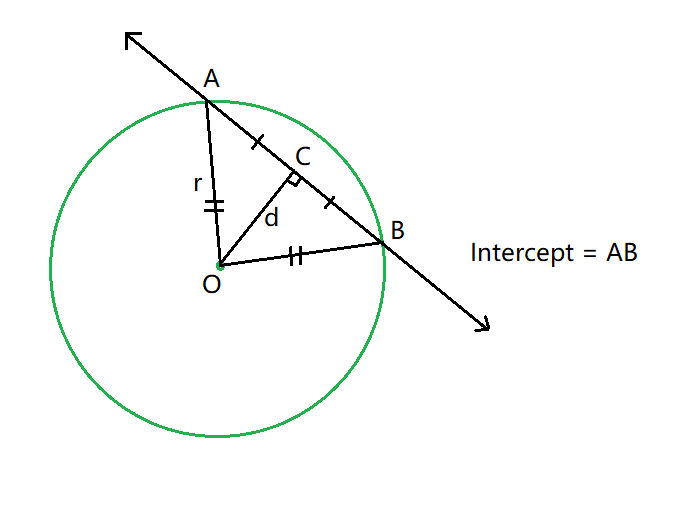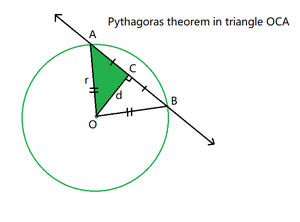Open In App

# Length of intercept cut off from a line by a Circle

Given six integers, a, b, c, i, j, and k representing the equation of the circleand equation of the line, the task is to find the length of the intercept cut off from the given line to the circle.Examples:

Input: a = 0, b = 0, c = -4, i = 2, j = -1, k = 1
Output: 3.89872

Input: a = 5, b = 6, c = -16, i = 1, j = 4, k = 3
Output: 6.9282

Approach: The problem can be solved based on the following mathematical fact.

As the line is intercepted by the circle the intercepted segment forms a chord of the circle. Now if a perpendicular is drawn from the centre of the circle to a chord, it divides the chord into two equal parts.

We can easily calculate the radius of the circle and the perpendicular distance of the chord from the centre from the given equations Using them we can get the length of the intercepted line segment.

Follow the steps below to solve the problem:

• Find the center of the circle, sayasand.
• The perpendicular from the center divides the intercept into two equal parts, therefore calculate the length of one of the parts and multiply it by 2 to get the total length of the intercept.
• Calculate the value of radius (r) using the formula:, whereand• Calculate the value of perpendicular distance ( d ) of center O from the line by using the formula:• Now from the Pythagoras theorem in triangle OCA:
•••• After completing the above steps, print the value of twice of AC to get the length of the total intercept.

Below is the implementation of the above approach.

## C++

 // C++ program for the above approach #include using namespace std; // Function to find the// radius of a circledouble radius(int a, int b, int c){    // g and f are the coordinates    // of the center    int g = a / 2;    int f = b / 2;     // Case of invalid circle    if (g * g + f * f - c < 0)        return (-1);     // Apply the radius formula    return (sqrt(g * g + f * f - c));} // Function to find the perpendicular// distance between circle center and the linedouble centerDistanceFromLine(int a, int b, int i, int j,                              int k){    // Store the coordinates of center    int g = a / 2;    int f = b / 2;     // Stores the perpendicular distance    // between the line and the point    double distance        = fabs(i * g + j * f + k) / (sqrt(i * i + j * j));     // Invalid Case    if (distance < 0)        return (-1);     // Return the distance    return distance;} // Function to find the length of intercept// cut off from a line by a circlevoid interceptLength(int a, int b, int c, int i, int j,                     int k){    // Calculate the value of radius    double rad = radius(a, b, c);     // Calculate the perpendicular distance    // between line and center    double dist = centerDistanceFromLine(a, b, i, j, k);     // Invalid Case    if (rad < 0 || dist < 0) {        cout << "circle not possible";        return;    }     // If line do not cut circle    if (dist > rad) {        cout << "Line not cutting circle";    }     // Print the intercept length    else        cout << 2 * sqrt(rad * rad - dist * dist);} // Driver Codeint main(){    // Given Input    int a = 0, b = 0, c = -4;    int i = 2, j = -1, k = 1;     // Function Call    interceptLength(a, b, c, i, j, k);     return 0;}

## Java

 // Java program for the above approachclass GFG{ // Function to find the// radius of a circlestatic double radius(int a, int b, int c){         // g and f are the coordinates    // of the center    int g = a / 2;    int f = b / 2;     // Case of invalid circle    if (g * g + f * f - c < 0)        return (-1);     // Apply the radius formula    return (Math.sqrt(g * g + f * f - c));} // Function to find the perpendicular// distance between circle center and the linestatic double centerDistanceFromLine(int a, int b,                                     int i, int j,                                     int k){         // Store the coordinates of center    int g = a / 2;    int f = b / 2;     // Stores the perpendicular distance    // between the line and the point    double distance = Math.abs(i * g + j * f + k) /                    (Math.sqrt(i * i + j * j));     // Invalid Case    if (distance < 0)        return (-1);     // Return the distance    return distance;} // Function to find the length of intercept// cut off from a line by a circlestatic void interceptLength(int a, int b, int c,                            int i, int j, int k){         // Calculate the value of radius    double rad = radius(a, b, c);     // Calculate the perpendicular distance    // between line and center    double dist = centerDistanceFromLine(        a, b, i, j, k);     // Invalid Case    if (rad < 0 || dist < 0)    {        System.out.println("circle not possible");        return;    }     // If line do not cut circle    if (dist > rad)    {        System.out.println("Line not cutting circle");    }     // Print the intercept length    else        System.out.println(2 * Math.sqrt(            rad * rad - dist * dist));} // Driver codepublic static void main(String[] args){         // Given Input    int a = 0, b = 0, c = -4;    int i = 2, j = -1, k = 1;     // Function Call    interceptLength(a, b, c, i, j, k);}} // This code is contributed by abhinavjain194

## Python3

 # Python3 program for the above approachimport math # Function to find the# radius of a circledef radius(a, b, c):     # g and f are the coordinates    # of the center    g = a / 2    f = b / 2     # Case of invalid circle    if (g * g + f * f - c < 0):        return(-1)     # Apply the radius formula    return(math.sqrt(g * g + f * f - c)) # Function to find the perpendicular# distance between circle center and the linedef centerDistanceFromLine(a, b, i, j, k):     # Store the coordinates of center    g = a / 2    f = b / 2     # Stores the perpendicular distance    # between the line and the point    distance = (abs(i * g + j * f + k) /         (math.sqrt(i * i + j * j)))     # Invalid Case    if (distance < 0):        return (-1)     # Return the distance    return distance # Function to find the length of intercept# cut off from a line by a circledef interceptLength(a, b, c, i, j, k):     # Calculate the value of radius    rad = radius(a, b, c)     # Calculate the perpendicular distance    # between line and center    dist = centerDistanceFromLine(        a, b, i, j, k)     # Invalid Case    if (rad < 0 or dist < 0):        print("circle not possible")        return     # If line do not cut circle    if (dist > rad):        print("Line not cutting circle")     # Print the intercept length    else:        print(2 * math.sqrt(            rad * rad - dist * dist)) # Driver Codeif __name__ == "__main__":     # Given Input    a = 0    b = 0    c = -4    i = 2    j = -1    k = 1     # Function Call    interceptLength(a, b, c, i, j, k) # This code is contributed by ukasp

## C#

 // C# program for the above approachusing System; class GFG{     // Function to find the// radius of a circlestatic double radius(int a, int b, int c){         // g and f are the coordinates    // of the center    int g = a / 2;    int f = b / 2;      // Case of invalid circle    if (g * g + f * f - c < 0)        return (-1);      // Apply the radius formula    return(Math.Sqrt(g * g + f * f - c));}  // Function to find the perpendicular// distance between circle center and the linestatic double centerDistanceFromLine(int a, int b,                                     int i, int j,                                     int k){         // Store the coordinates of center    int g = a / 2;    int f = b / 2;      // Stores the perpendicular distance    // between the line and the point    double distance = Math.Abs(i * g + j * f + k) /                    (Math.Sqrt(i * i + j * j));      // Invalid Case    if (distance < 0)        return (-1);      // Return the distance    return distance;}  // Function to find the length of intercept// cut off from a line by a circlestatic void interceptLength(int a, int b, int c,                            int i, int j, int k){          // Calculate the value of radius    double rad = radius(a, b, c);      // Calculate the perpendicular distance    // between line and center    double dist = centerDistanceFromLine(        a, b, i, j, k);      // Invalid Case    if (rad < 0 || dist < 0)    {        Console.WriteLine("circle not possible");        return;    }      // If line do not cut circle    if (dist > rad)    {        Console.WriteLine("Line not cutting circle");    }      // Print the intercept length    else        Console.WriteLine(2 * Math.Sqrt(            rad * rad - dist * dist));} // Driver codepublic static void Main(String []args){         // Given Input    int a = 0, b = 0, c = -4;    int i = 2, j = -1, k = 1;      // Function Call    interceptLength(a, b, c, i, j, k);}} // This code is contributed by sanjoy_62

## Javascript

 

Output

3.89872

Time Complexity: O(logN), for using in-built sqrt() function.
Auxiliary Space: O(1)